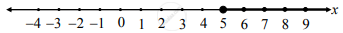# Sketch the graph which represents the solution set for the following inequation.x ≥ 5 - Mathematics and Statistics

Graph

Sketch the graph which represents the solution set for the following inequation.
x ≥ 5

#### Solution

x ≥ 5
Here, x takes all real values that are greater than or equal to 5
∴ Solution set represents the unbounded (semi-left closed) interval (5, ∞)
∴ the required graph of the solution set is as follows:Concept: Graphical Representation of Solution of Linear Inequality in One Variable
Is there an error in this question or solution?

#### APPEARS IN

Balbharati Mathematics and Statistics 2 (Commerce) 11th Standard HSC Maharashtra State Board
Chapter 8 Linear Inequations
Exercise 8.1 | Q 3. (ii) | Page 116